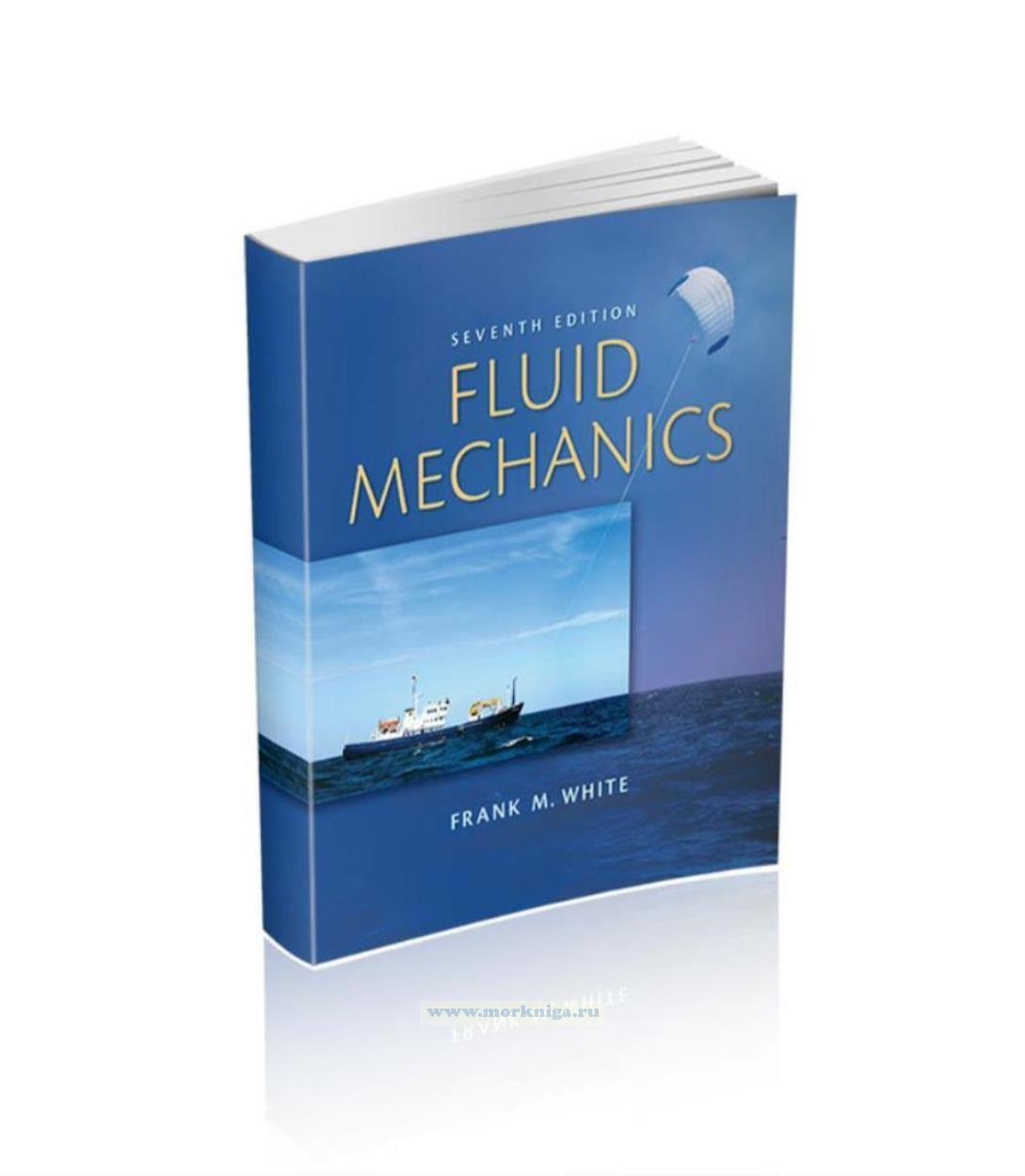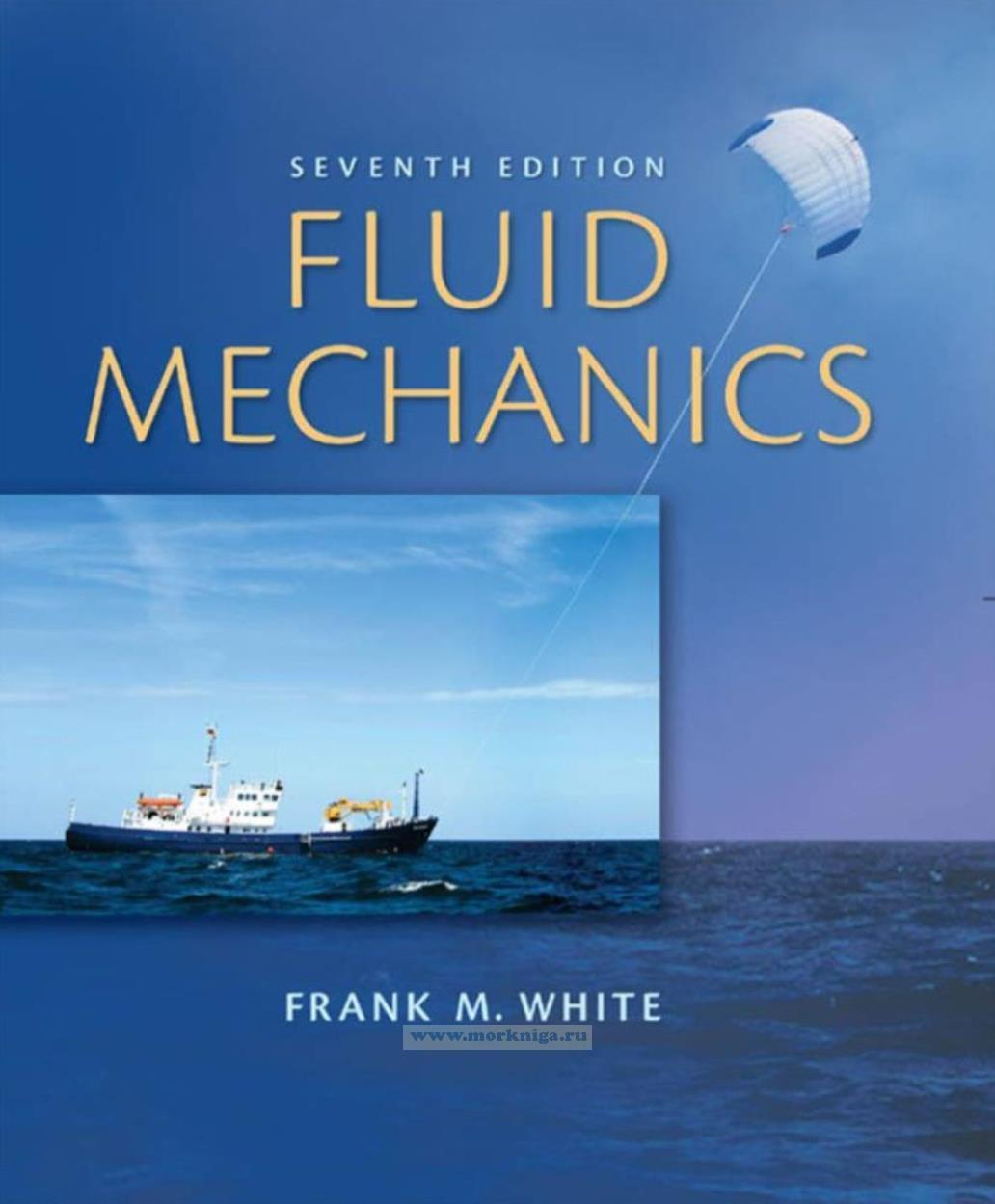звоните нам Пн-Пт с 9 до 19,
Сб с 10 до 16
Каталог

# Fluid Mechanics. Seventh Edition/ Механика жидкостей. Седьмое издание

••Артикул: 00-01024911
в желания В наличии
Автор: Frank M. White
Издательство: McGraw Hill (все книги издательства)
ISBN: 978-0-07-352934-9
Год: 2011
Переплет: Мягкая обложка
Страниц: 885
Вес: 2206 г
5900 P
-
+
С этим товаром покупают

Издание на английском языке
The seventh edition of Fluid Mechanics sees some additions and deletions but no philosophical change. The basic outline of eleven chapters, plus appendices, remains the same. The triad of integral, differential, and experimental approaches is retained.
Many problem exercises, and some fully worked examples, have been changed. The informal, student-oriented style is retained. A number of new photographs and figures have been added. Many new references have been added, for a total of 435. The writer is a firm believer in “further reading,” especially in the postgraduate years.
The total number of problem exercises continues to increase, from 1089 in the first edition, to 1675 in this seventh edition. There are approximately 20 new problems added to each chapter. Most of these are basic end-of-chapter problems, classified according to topic. There are also Word Problems, multiple-choice Fundamentals of Engineering Problems, Comprehensive Problems, and Design Projects. The appendix lists approximately 700 Answers to Selected Problems.
The example problems are structured in the text to follow the sequence of recommended steps outlined in Sect. 1.3, Problem-Solving Techniques.
The Engineering Equation Solver (EES) is available with the text and continues its role as an attractive tool for fluid mechanics and, indeed, other engineering problems.
Not only is it an excellent solver, but it also contains thermophysical properties, publication-quality plotting, units checking, and many mathematical functions, including numerical integration.

Contents
Preface
Chapter 1 Introduction
1.1 Preliminary Remarks
1.2 History and Scope of Fluid Mechanics
1.3 Problem-Solving Techniques
1.4 The Concept of a Fluid
1.5 The Fluid as a Continuum
1.6 Dimensions and Units
1.7 Properties of the Velocity Field
1.8 Thermodynamic Properties of a Fluid
1.9 Viscosity and Other Secondary Properties
1.10 Basic Flow Analysis Techniques
1.11 Flow Patterns: Streamlines, Streaklines, and Pathlines
1.12 The Engineering Equation Solver
1.13 Uncertainty in Experimental Data
1.14 The Fundamentals of Engineering (FE)
Examination
Problems
Fundamentals of Engineering Exam Problems
Comprehensive Problems
References
Chapter 2 Pressure Distribution in a Fluid
2.2 Equilibrium of a Fluid Element
2.3 Hydrostatic Pressure Distributions
2.4 Application to Manometry
2.5 Hydrostatic Forces on Plane Surfaces
2.6 Hydrostatic Forces on Curved Surfaces
2.7 Hydrostatic Forces in Layered Fluids
2.8 Buoyancy and Stability
2.9 Pressure Distribution in Rigid-Body Motion
2.10 Pressure Measurement
Summary
Problems
Word Problems
Fundamentals of Engineering Exam
Problems
Comprehensive Problems
Design Projects
References
Chapter 3 Integral Relations for a Control Volume
3.1 Basic Physical Laws of Fluid Mechanics
3.2 The Reynolds Transport Theorem
3.3 Conservation of Mass
3.4 The Linear Momentum Equation
3.5 Frictionless Flow: The Bernoulli Equation
3.6 The Angular Momentum Theorem
3.7 The Energy Equation
Summary
Problems
Word Problems
Fundamentals of Engineering Exam Problems
Comprehensive Problems
Design Project
References
Chapter 4 Differential Relations for Fluid Flow
4.1 The Acceleration Field of a Fluid
4.2 The Differential Equation of Mass Conservation
4.3 The Differential Equation of Linear Momentum
4.4 The Differential Equation of Angular Momentum
4.5 The Differential Equation of Energy
4.6 Boundary Conditions for the Basic Equations
4.7 The Stream Function
4.8 Vorticity and Irrotationality
4.9 Frictionless Irrotational Flows
4.10 Some Illustrative Incompressible Viscous Flows
Summary
Problems
Word Problems
Fundamentals of Engineering Exam Problems
Comprehensive Problems
References
Chapter 5 Dimensional Analysis and Similarity
5.1 Introduction
5.2 The Principle of Dimensional Homogeneity
5.3 The Pi Theorem
5.4 Nondimensionalization of the Basic Equations
5.5 Modeling and Its Pitfalls
Summary
Problems
Word Problems
Fundamentals of Engineering Exam Problems
Comprehensive Problems
Design Projects
References
Chapter 6 Viscous Flow in Ducts
6.1 Reynolds Number Regimes
6.2 Internal versus External Viscous Flow
6.4 Laminar Fully Developed Pipe Flow
6.5 Turbulence Modeling
6.6 Turbulent Pipe Flow
6.7 Four Types of Pipe Flow Problems
6.8 Flow in Noncircular Ducts
6.9 Minor or Local Losses in Pipe Systems
6.10 Multiple-Pipe Systems
6.11 Experimental Duct Flows: Diffuser Performance
6.12 Fluid Meters
Summary
Problems
Word Problems
Fundamentals of Engineering Exam Problems
Comprehensive Problems
Design Projects
References
Chapter 7 Flow Past Immersed Bodies
7.1 Reynolds Number and Geometry Effects
7.2 Momentum Integral Estimates
7.3 The Boundary Layer Equations
7.4 The Flat-Plate Boundary Layer
7.5 Boundary Layers with Pressure Gradient
7.6 Experimental External Flows
Summary
Problems
Word Problems
Fundamentals of Engineering Exam Problems
Comprehensive Problems
Design Project
References
Chapter 8 Potential Flow and Computational Fluid Dynamics
8.1 Introduction and Review
8.2 Elementary Plane Flow Solutions
8.3 Superposition of Plane Flow Solutions
8.4 Plane Flow Past Closed-Body Shapes
8.5 Other Plane Potential Flows
8.6 Images
8.7 Airfoil Theory
8.8 Axisymmetric Potential Flow
8.9 Numerical Analysis
Summary
Problems
Word Problems
Comprehensive Problems
Design Projects
References
Chapter 9 Compressible Flow
9.1 Introduction: Review of Thermodynamics
9.2 The Speed of Sound
9.4 Isentropic Flow with Area Changes
9.5 The Normal Shock Wave
9.6 Operation of Converging and Diverging Nozzles
9.7 Compressible Duct Flow with Friction
9.8 Frictionless Duct Flow with Heat Transfer
9.9 Two-Dimensional Supersonic Flow
9.10 Prandtl-Meyer Expansion Waves
Summary
Problems
Word Problems
Fundamentals of Engineering Exam Problems
Comprehensive Problems
Design Projects
References
Chapter 10 Open-Channel Flow
10.1 Introduction
10.2 Uniform Flow: The Ch?zy Formula
10.3 Efficient Uniform-Flow Channels
10.4 Specific Energy: Critical Depth
10.5 The Hydraulic Jump
10.7 Flow Measurement and Control by Weirs
Summary
Problems
Word Problems
Fundamentals of Engineering Exam Problems
Comprehensive Problems
Design Projects
References
Chapter 11 Turbomachinery
11.1 Introduction and Classification
11.2 The Centrifugal Pump
11.3 Pump Performance Curves and Similarity Rules
11.4 Mixed- and Axial-Flow Pumps: The Specific Speed
11.5 Matching Pumps to System Characteristics
11.6 Turbines
Summary
Problems
Word Problems
Comprehensive Problem
Design Project
References
Appendix A Physical Properties of Fluids
Appendix B Compressible Flow Tables
Appendix C Conversion Factors
Appendix D Equations of Motion in Cylindrical Coordinates